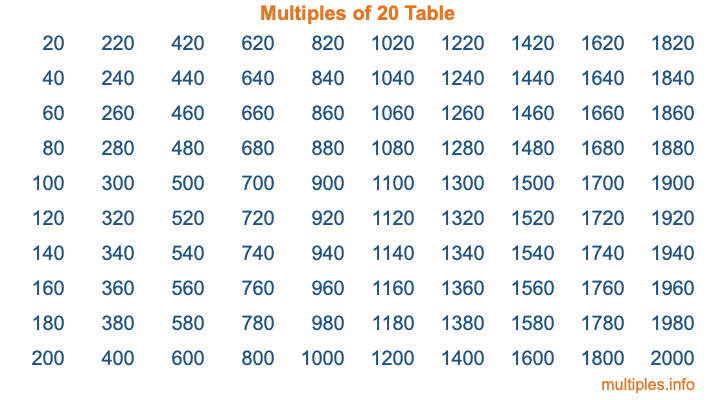Multiples of 20Welcome to the Multiples of 20 page. Here we will first teach you everything you will ever need to know about the multiples of 20, and then give you a study guide summary of everything we taught you to make sure you remember it all. Use this page to look up facts and learn information about the multiples of 20. This page will make you a multiples of twenty expert!

Definition of Multiples of 20
Multiples of 20 are all the numbers that when divided by 20 equal an integer. Each of the multiples of 20 are called a multiple. A multiple of 20 is created by multiplying 20 by an integer.

Therefore, to create a list of multiples of 20, you start with 1 multiplied by 20, then 2 multiplied by 20, then 3 multiplied by 20, and so on for as long as you want. Thus, the list of the first five multiples of 20 is 20, 40, 60, 80, and 100. To see a larger list of multiples of 20, see the printable image of Multiples of 20 further down on this page. We also have a category where you can choose any nth multiple of 20.

Multiples of 20 Checker
The Multiples of 20 Checker below checks to see if any number of your choice is a multiple of 20. In other words, it checks to see if there is any number (integer) that when multiplied by 20 will equal your number. To do that, we divide your number by 20. If the the quotient is an integer, then your number is a multiple of 20.

Is  a multiple of 20?

Least Common Multiple of 20 and ...
A Least Common Multiple (LCM) is the lowest multiple that two or more numbers have in common. This is also called the smallest common multiple or lowest common multiple and is useful to know when you are adding our subtracting fractions. Enter one or more numbers below (20 is already entered) to find the LCM.

Check out our LCM Calculator if you need more details about the Least Common Multiple or if you need the LCM for different numbers for adding and subtraction fractions.

nth Multiple of 20
As we stated above, 20 is the first multiple of 20, 40 is the second multiple of 20, 60 is the third multiple of 20, and so on. Enter a number below to find the nth multiple of 20.

th multiple of 20

Multiples of 20 vs Factors of 20
20 is a multiple of 20 and a factor of 20, but that is where the similarities end. All postive multiples of 20 are 20 or greater than 20. All positive factors of 20 are 20 or less than 20.

Below is the beginning list of multiples of 20 and the factors of 20 so you can compare:

Multiples of 20: 20, 40, 60, 80, 100, etc.

Factors of 20: 1, 2, 4, 5, 10, 20

As you can see, the multiples of 20 are all the numbers that you can divide by 20 to get a whole number. The factors of 20, on the other hand, are all the whole numbers that you can multiply by another whole number to get 20.

It's also interesting to note that if a number (x) is a factor of 20, then 20 will also be a multiple of that number (x).

Multiples of 20 vs Divisors of 20
The divisors of 20 are all the integers that 20 can be divided by evenly. Below is a list of the divisors of 20.

Divisors of 20: 1, 2, 4, 5, 10, 20

The interesting thing to note here is that if you take any multiple of 20 and divide it by a divisor of 20, you will see that the quotient is an integer.

Multiples of 20 Table
Below is an image of the first 100 multiples of 20 in a table. The table is in chronological order, column by column. The first column has the first ten multiples of 20, the second column has the next ten multiples of 20, and so on.The Multiples of 20 Table is also referred to as the 20 Times Table or Times Table of 20. You are welcome to print out our table for your studies.

Negative Multiples of 20
Although not often discussed or needed in math, it is worth mentioning that you can make a list of negative multiples of 20 by multiplying 20 by -1, then by -2, then by -3, and so on, to get the following list of negative multiples of 20:

-20, -40, -60, -80, -100, etc.

Multiples of 20 Summary
Below is a summary of important Multiples of 20 facts that we have discussed on this page. To retain the knowledge on this page, we recommend that you read through the summary and explain to yourself or a study partner why they hold true.

There are an infinite number of multiples of 20.

A multiple of 20 divided by 20 will equal a whole number.

20 divided by a factor of 20 equals a divisor of 20.

The nth multiple of 20 is n times 20.

The largest factor of 20 is equal to the first positive multiple of 20.

20 is a multiple of every factor of 20.

20 is a multiple of 20.

A multiple of 20 divided by a divisor of 20 equals an integer.

20 divided by a divisor of 20 equals a factor of 20.

Any integer times 20 will equal a multiple of 20.

Multiples of a Number
Here you can get the multiples of another number, all with the same attention to detail as we did for multiples of 20 on this page.

Multiples of
Multiples of 21
Did you find our page about multiples of twenty educational? Do you want more knowledge? Check out the multiples of the next number on our list!